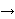## Genus of Paley graphs

Let q be a power of a prime, q = pk, where p1 (mod 4). The Paley graph Pq is the Cayley graph of the additive group GF(q) generated with all squares. More precisely, V(Pq) = GF(q), and vertices x, y are adjacent if x - y = z2 for some z in GF(q)\{0}. Since q1 (mod 4),  x - y is a square if and only if y - x is a square.

Paley graphs have a number of intriguing properties. On one hand, they are highly symmetric - their automorphism group acts transitively on the vertices, and they are self-complementary. On the other hand, they resemble random graphs a lot.

Problem 1: Determine the genus of  Pq.

It seems plausible to expect that Paley graphs admit embeddings that are close to be triangular. In other words, we expect the genus of  Pq to be close to (q2 - 13q + 24)/24. In a special case, we dare to conjecture that this is the case:

Conjecture 1: If q is a square, then the genus of Pq is (q2 - 13q + 24)(1/24 + o(1)), where o(1)0 when q increases.

It is known (cf. ) that every Paley graph Pq with q1 (mod 8) admits an embedding in the orientable surface of genus (q-1)(q-8)/8 which is flag-transitive and, surprisingly enough, self-dual.

Bibliography:

 A.T. White, Graphs of groups on surfaces. Interactions and models. North-Holland Mathematics Studies 188, Amsterdam, 2001.